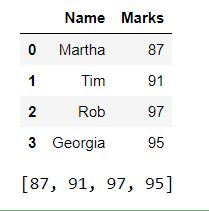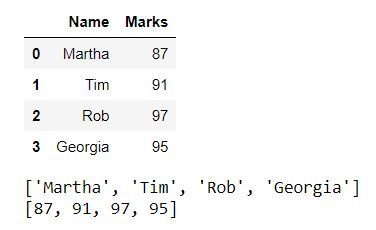# Get a list of a particular column values of a Pandas DataFrame

In this article, we’ll see how to get all values of a column in a pandas dataframe in the form of a list. This can be very useful in many situations, suppose we have to get marks of all the students in a particular subject, get phone numbers of all employees, etc. Let’s see how we can achieve this with the help of some examples.

Example 1: We can have all values of a column in a list, by using the tolist() method.

Syntax: Series.tolist().

Return type: Converted series into List.

Code:

## Python3

 `# import pandas libraey ` `import` `pandas as pd ` ` `  `# dictionary ` `dict` `=` `{``'Name'``: [``'Martha'``, ``'Tim'``, ` `                ``'Rob'``, ``'Georgia'``], ` `         ``'Marks'``: [``87``, ``91``,  ` `                  ``97``, ``95``]} ` ` `  `# create a dataframe object ` `df ``=` `pd.DataFrame(``dict``) ` ` `  `# show the dataframe ` `print``(df) ` ` `  `# list of values of 'Marks' column ` `marks_list ``=` `df[``'Marks'``].tolist() ` ` `  `# show the list ` `print``(marks_list)`

Output:Example 2: We’ll see how we can get the values of all columns in separate lists.

Code:

## Python3

 `# import pandas library ` `import` `pandas as pd ` ` `  `# dictionary ` `dict` `=` `{``'Name'``: [``'Martha'``, ``'Tim'``,  ` `                ``'Rob'``, ``'Georgia'``], ` `        ``'Marks'``: [``87``, ``91``,  ` `                 ``97``, ``95``]} ` ` `  `# create a dataframe object ` `df ``=` `pd.DataFrame(``dict``) ` ` `  `# show the dataframe ` `print``(df) ` ` `  `# iterating over and calling  ` `# tolist() method for  ` `# each column ` `for` `i ``in` `list``(df): ` `   `  `    ``# show the list of values   ` `    ``print``(df[i].tolist()) `

Output:My Personal Notes arrow_drop_upCheck out this Author's contributed articles.

If you like GeeksforGeeks and would like to contribute, you can also write an article using contribute.geeksforgeeks.org or mail your article to contribute@geeksforgeeks.org. See your article appearing on the GeeksforGeeks main page and help other Geeks.

Please Improve this article if you find anything incorrect by clicking on the "Improve Article" button below.

Article Tags :

1

Please write to us at contribute@geeksforgeeks.org to report any issue with the above content.